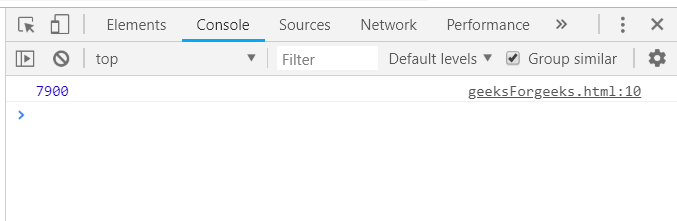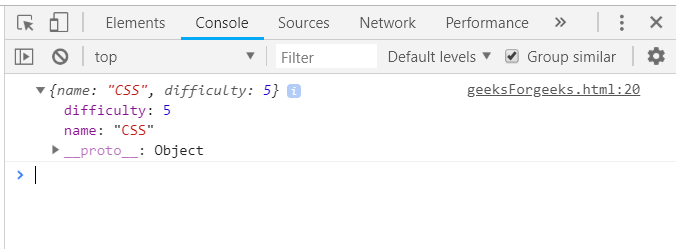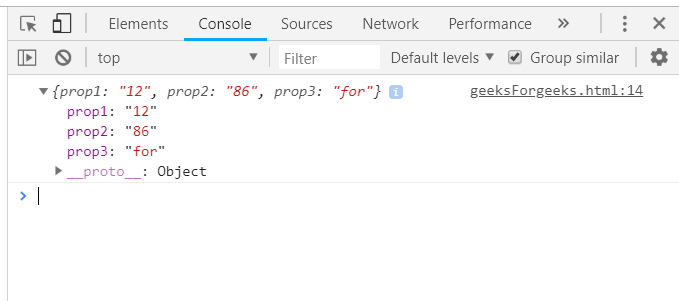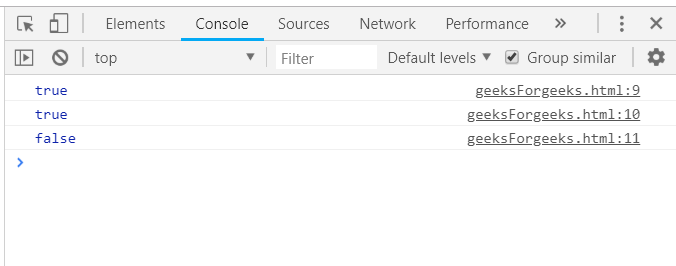GeeksforGeeks App
Open AppBrowser
Continue

# Underscore.js _.max Function

The Underscore.js is a JavaScript library that provides a lot of useful functions that help in programming in a big way like the map, filter, invokes, etc even without using any built-in objects. The _.max() function is used to find the minimum element from the list passed. If an iterate is given, then it will be applied to each value and generate criteria to rank the values and find the minimum element.

Syntax:

`_.max(list, [iterate], [context])`

Parameters: This function accepts three parameters as mentioned above and described below:

• List: This parameter is used to hold the list of items.
• Predicate: This parameter is used to hold the test condition.
• Context: This parameter is used to display the content.

Return values: The return value is the element of the list that is maximum. A numbers list will give the maximum number and that of the string will give the string which is last when they are placed alphabetically.

Passing an array of numbers to the _.max function(): The ._max() function takes the element from the list one by one and do compare the elements to find the maximum number in the list. After traversing and comparing all the elements, the _.max() function ends.

Example:

## html

 `<``html``>``    ``<``head``>``        ``<``script` `type="text/javascript" src =``"https://cdnjs.cloudflare.com/ajax/libs/underscore.js/1.9.1/underscore-min.js" >``        ````    ````    ``<``body``>``        ``<``script` `type="text/javascript">``            ``var numbers = [100, 50, 400, 66, 7900];``            ``console.log(_.max(numbers));``        ````    `````

Output:Passing a list of both numbers and strings as its property to _.max() function: Passing a list of both numbers and strings and comparing the elements by one of the properties. Either by the property of the number or by the string property. Here we are comparing the ‘difficulty’ property. The largest difficulty element will be returned.

Example:

## html

 `<``html``>``    ``<``head``>``        ``<``script` `type="text/javascript" src = "https://cdnjs.cloudflare.com/ajax/libs/underscore.js/1.9.1/underscore-min.js" >``        ````    ````    ``<``body``>``        ``<``script` `type="text/javascript">``             ``var languages = [``                    ``{``                    ``name: 'HTML',``                    ``difficulty: 4``                    ``},``                    ``{``                    ``name: 'CSS',``                    ``difficulty: 5``                    ``}``                ``];``            ``console.log(_.max(languages, function(o)``            ``{``                ``return o.difficulty;``            ``}));``        ````    `````

Output:Passing a structure of more than 1 property to the _.max() function: First, declare the array (here array is ‘arr’) and choose one property out of the many, on the basis of which need to find the maximum like here ‘hasLongHairs’. Console.log is the variable in which this returned maximum value is stored.

Example:

## html

 `<``html``>``    ``<``head``>``        ``<``script` `type="text/javascript" src =``"https://cdnjs.cloudflare.com/ajax/libs/underscore.js/1.9.1/underscore-min.js" >``        ````    ````    ``<``body``>``        ``<``script` `type="text/javascript">``             ``var arr =  [``                ``{prop1:"10", prop2:"07", prop3: "Geeks"},``                ``{prop1:"12", prop2:"86", prop3: "for"},``                ``{prop1:"11", prop2:"58", prop3: "Geeks."}``            ``];``            ``console.log(_.max(arr, function(o){return o.prop2;}));``        ````    `````

Output:Passing ‘true’ and ‘false’ as the elements of the list to the _.max() function: Passing the ‘true’ and ‘false’ values to the _.max() function. The maximum of these values will be defined as ‘true’ if it is present in the list at least once otherwise answer will be ‘false’. This is just the opposite if the ‘_.min()’ function.

Example:

## html

 `<``html``>``    ``<``head``>``        ``<``script` `type="text/javascript" src =``"https://cdnjs.cloudflare.com/ajax/libs/underscore.js/1.9.1/underscore-min.js" >``        ````    ````    ``<``body``>``        ``<``script` `type="text/javascript">``           ``console.log(_.max([true, false, true]));``           ``console.log(_.max([true, true]));``           ``console.log(_.max([false, false]));``        ````    `````

Output:My Personal Notes arrow_drop_up
Related Tutorials